GFG App
Open AppBrowser
Continue

# Types of Trees in Data Structures

## What is a Tree?

A Tree is a non-linear data structure and a hierarchy consisting of a collection of nodes such that each node of the tree stores a value and a list of references to other nodes (the “children”).

## Types of Trees in Data Structure based on the number of children: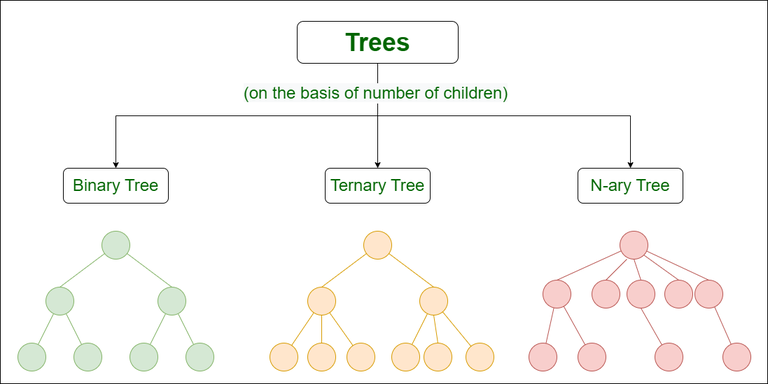Types of Trees in Data Structure based on the number of children

To know more about the Special Types of Trees, please refer to this detailed article: Types of Binary Tree.

Let us see about these trees one by one:

## 1. Binary Tree

A binary Tree is defined as a Tree data structure with at most 2 children. Since each element in a binary tree can have only 2 children, we typically name them the left and right child.

Example:
Consider the tree below. Since each node of this tree has only 2 children, it can be said that this tree is a Binary Tree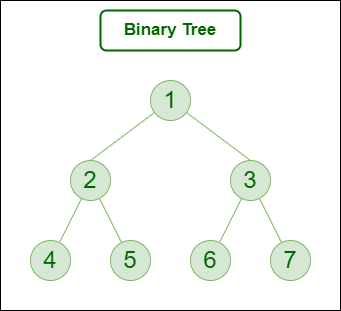Binary Tree

### Types of Binary Tree:

• Binary Tree consists of following types based on the  number of children:
1. Full Binary Tree
2. Degenerate Binary Tree
• On the basis of completion of levels, the binary tree can be divided into following types:

To know more about the types of the binary trees, please refer to this detailed article: Types of Binary Tree.

## 2. Ternary Tree

A Ternary Tree is a tree data structure in which each node has at most three child nodes, usually distinguished as “left”, “mid” and “right”.

Example:
Consider the tree below. Since each node of this tree has only 3 children, it can be said that this tree is a Ternary Tree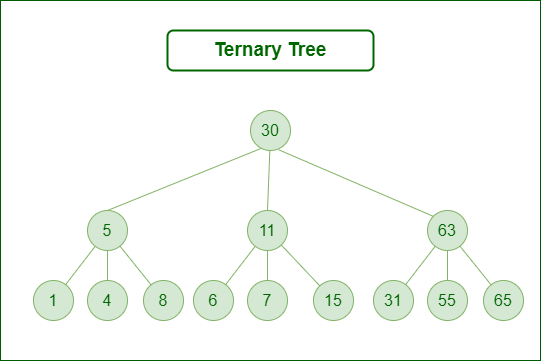Ternary Tree

### Types of Ternary Tree:

Ternary Search Tree
A ternary search tree is a special trie data structure where the child nodes of a standard trie are ordered as a binary search tree.

Unlike trie(standard) data structure where each node contains 26 pointers for its children, each node in a ternary search tree contains only 3 pointers:
1. The left pointer points to the node whose value is less than the value in the current node.
2. The equal pointer points to the node whose value is equal to the value in the current node.
3. The right pointer points to the node whose value is greater than the value in the current node.

## 3. N-ary Tree (Generic Tree)

Generic trees are a collection of nodes where each node is a data structure that consists of records and a list of references to its children(duplicate references are not allowed). Unlike the linked list, each node stores the address of multiple nodes.

Every node stores the addresses of its children and the very first node’s address will be stored in a separate pointer called root.

1. Many children at every node.
2. The number of nodes for each node is not known in advance.

Example: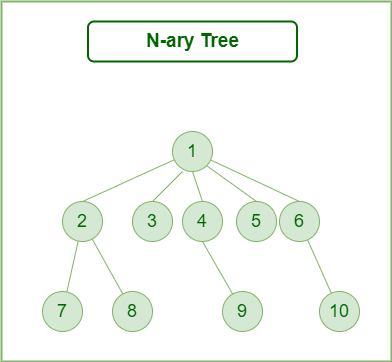N-ary Tree

To know more about N-ary Tree, please refer to this detailed article: N-ary Tree

## 1. Binary Search Tree

A binary Search Tree is a node-based binary tree data structure that has the following properties:

• The left subtree of a node contains only nodes with keys lesser than the node’s key.
• The right subtree of a node contains only nodes with keys greater than the node’s key.
• The left and right subtree each must also be a binary search tree.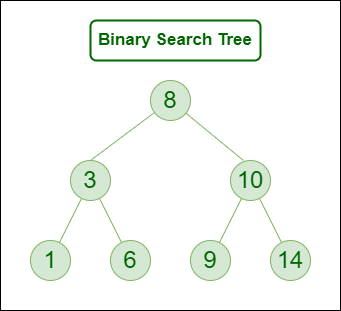Binary Search Tree

## 2. AVL Tree

AVL tree is a self-balancing Binary Search Tree (BST) where the difference between heights of left and right subtrees for any node cannot be more than one.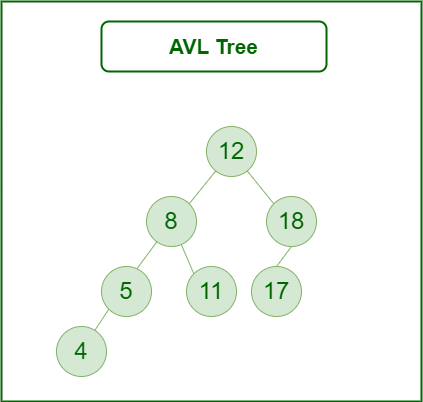AVL Tree

## 3. Red-Black Tree

A red-black tree is a kind of self-balancing binary search tree where each node has an extra bit, and that bit is often interpreted as the color (red or black). These colors are used to ensure that the tree remains balanced during insertions and deletions.

Although the balance of the tree is not perfect, it is good enough to reduce the searching time and maintain it around O(log n) time, where n is the total number of elements in the tree.

#### Rules That Every Red-Black Tree Follows:

1. Every node has a color either red or black.
2. The root of the tree is always black.
3. There are no two adjacent red nodes (A red node cannot have a red parent or red child).
4. Every path from a node (including root) to any of its descendants’ NULL nodes has the same number of black nodes.
5. All leaf nodes are black nodes.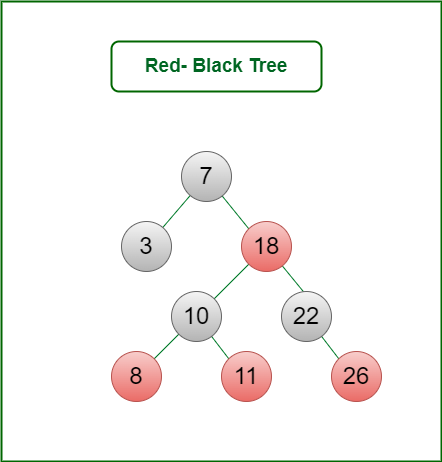Red-Black Tree

## 4. B-Tree

B-Tree is a self-balancing search tree. In most of the other self-balancing search trees (like AVL and Red-Black Trees), it is assumed that everything is in the main memory.

## Properties of B-Tree:

• All leaves are at the same level.
• B-Tree is defined by the term minimum degree ‘t‘. The value of ‘t‘ depends upon disk block size.
• Every node except the root must contain at least t-1 keys. The root may contain a minimum of 1 key.
• All nodes (including root) may contain at most (2*t – 1) keys.
• The number of children of a node is equal to the number of keys in it plus 1.
• All keys of a node are sorted in increasing order. The child between two keys k1 and k2 contains all keys in the range from k1 and k2.
• B-Tree grows and shrinks from the root which is unlike Binary Search Tree. Binary Search Trees grow downward and also shrink from downward.
• Like other balanced Binary Search Trees, the time complexity to search, insert and delete is O(log n).
• Insertion of a Node in B-Tree happens only at Leaf Node.

## 5. B+ Tree

B+ tree eliminates the drawback B-tree used for indexing by storing data pointers only at the leaf nodes of the tree. Thus, the structure of leaf nodes of a B+ tree is quite different from the structure of internal nodes of the B tree.

It may be noted here that, since data pointers are present only at the leaf nodes, the leaf nodes must necessarily store all the key values along with their corresponding data pointers to the disk file block, to access them. Moreover, the leaf nodes are linked to providing ordered access to the records. The leaf nodes, therefore form the first level of the index, with the internal nodes forming the other levels of a multilevel index. Some of the key values of the leaf nodes also appear in the internal nodes, to simply act as a medium to control the search of a record.

The structure of the internal nodes of a B+ tree of order ‘a’ is as follows:

1. Each internal node is of the form: <P1, K1, P2, K2, ….., Pc-1, Kc-1, Pc> where c <= a and each Pi is a tree pointer (i.e points to another node of the tree) and, each Ki is a key-value (see diagram-I for reference).
2. Every internal node has : K1 < K2 < …. < Kc-1
3. For each search field values ‘X’ in the sub-tree pointed at by Pi, the following condition holds: Ki-1 < X <= Ki, for 1 < i < c and, Ki-1 < X, for i = c (See diagram I for reference)
4. Each internal node has at most ‘a’ tree pointers.
5. The root node has, at least two tree pointers, while the other internal nodes have at least \ceil(a/2) tree pointers each.
6. If an internal node has ‘c’ pointers, c <= a, then it has ‘c – 1’ key values.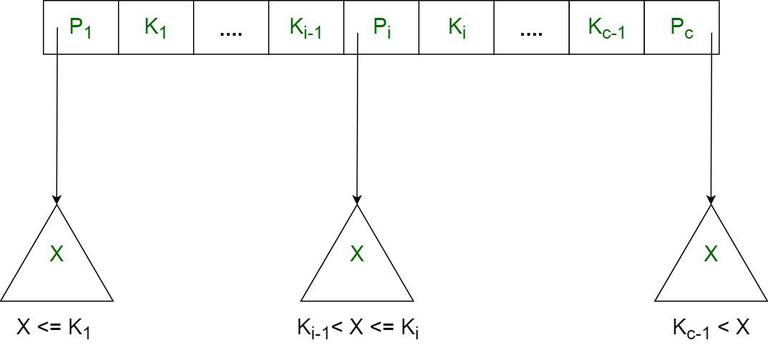B+ Tree

## 6. Segment Tree

In computer science, a Segment Tree, also known as a statistic tree, is a tree data structure used for storing information about intervals, or segments. It allows querying which of the stored segments contain a given point. It is, in principle, a static structure; that is, it’s a structure that cannot be modified once it’s built. A similar data structure is the interval tree.

A segment tree for a set I of n intervals uses O(n log n) storage and can be built in O(n log n) time. Segment trees support searching for all the intervals that contain a query point in time O(log n + k), k being the number of retrieved intervals or segments.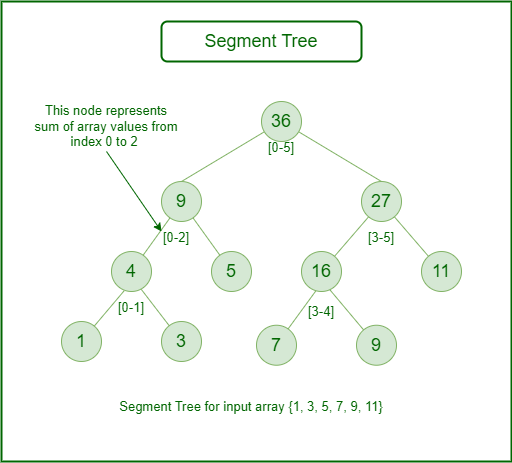Segment Tree

Related Articles:

My Personal Notes arrow_drop_up